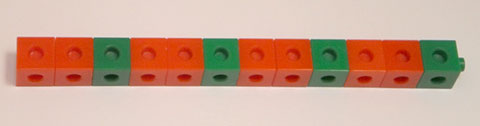Home > Patterns > Big ideas > Repeating patterns > Repeating patterns and multiplication

# Repeating patterns and multiplication

Exploration of repeating patterns can form an easy introduction to some of the fundamental ideas of early mathematics.

Here is a very simple repeating pattern made from coloured blocks. It is called a 'train'.An AAB repeating pattern.

• The number of blocks is 1, 2, 3; 4, 5, 6; 7, 8, 9; 10, 11, 12 (rhythmic counting).
• The number of blocks is 3, 6, 9, 12 (skip counting).
• There are 4 lots of 3 blocks, making 12 blocks in all.
• Each unit of repeat is a quarter of the whole object.

After exploring many other similar trains, students could reach some more general ideas.

• To find the number of blocks in a train, you multiply the number of blocks in the unit of repeat by the number of repetitions.
• To find the number of repetitions needed to make a train of a given size, you divide the total number of blocks by the number of blocks in the unit of repeat.
• A number is even if you can make a train with that number of blocks using a unit of repeat consisting of two blocks. If not, the number is odd.

Can you see any other general ideas here?

Year 1: Develop confidence with number sequences to and from 100 by ones from any starting point. Skip count by twos, fives and tens starting from zero

Year 2: Recognise and represent multiplication as repeated addition, groups and arrays

Year 2: Recognise and represent division as grouping into equal sets and solve simple problems using these representations

Year 3: Investigate the conditions required for a number to be odd or even and identify odd and even numbers

Year 3: Recall multiplication facts of two, three, five and ten and related division facts

Year 3: Model and represent unit fractions including 1/2, 1/4, 1/3, 1/5 and their multiples to a complete whole

Source